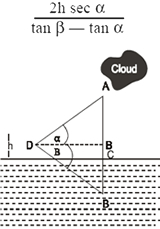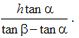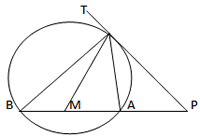Sunday 31st May 2020CBSE Guess > Papers > Important Questions > Class X > 2014 > Mathematics > Math-index > Mathematics By Mr. Ajai Kumar Shukla CBSE CLASS X Mathematics MATHEMATICS Determine the ratio in which the points P (m, 6) divides the join of A (-4, 3) and B (2, 8). Also find the value of m. Find the value of p for which the points (-1, 3), (2, p) and (5,-1) are collinear. In given figure, ABCD is a square whose each side is 14 cm. APD and BPC are semicircles. Find the area of the shaded regionThe sum of the digits of two digits number is 9. Also, nine times this number is twice the number obtained by reversing the order of the digits of the number Find the number. A motor boat whose speed is 15km/hr in still water , goes 30km downstream and comes back in a total time of 4 hours 30 minutes. Find the stream State and prove Pythagoras Theorem. Using it, if ABC is a triangle in which AB = AC and D is any point in BC, Prove that: AB2--AD2=BD.CD If the angle of elevation of a cloud from a point h meters above a lake is α and the angle of depression of its reflection in the lake is b, prove that the distance of the cloud from the point of observation isThe length of a tangent from a point A at a distance of 5 cm from the center of the circle is 4 cm. What will be the radius of the circle? A card is drawn at random from a well-shuffled deck of 52 cards.Find the probability that it is neither an ace nor a queen. The minute hand of a clock is 10 cm. long. Find the area on the face of the clock described by the minute hand in10 minutes. From the top of a church spire 96 m high the angles of depression of two vehicles on a road, at the same level as the base of the spire and on the same side of it are x° and y°, where tan x° = 1/4 and tan y° = 1/7. Calculate the distance between the vehicles. Water flows out through a circular pipe, whose internal diameter is 2cm, at the rate of 0.7m per second into a cylindrical tank, the radius of whose base is 4cm. By how much the water level will rise. A conical vessel of radius 6 cm and height 8 cm is completely filled with water. A sphere is lowered in to water and its size is such that when it touches the sides, it is just immersed. What fraction of water overflows? If the point (x, y) is equidistant from the points (a +b, b-a) and (a-b, a+b,)prove that b x =ay. Determine the ratio in which the point (-6,a) divides the join of A(-3,-1) and B(-8,9). Also find the value of a. Find the area of the triangle ABC formed by joining the mid-points of the sides of the triangle whose vertices are A( 4, -6 ), B( 3, -2 ) and C ( 5, 2 ). Determine the ratio in which the point (-6,a) divides the join of A(-3,-1) and B(-8,9). Also find the value of a. A vertical tower is surmounted by a flagstaff of height h meters. At a point on the ground, the angles of elevation of the bottom and top of the flagstaff are a and b respectively. Prove that height of the tower isIn fig PT is a tangent and PAB is a secant of the circle. If the bisector of LATB  Intersects  AB at M, Prove that PT = PMA vessel is in the form of an inverted cone which is open at the top. Its depth is 8cm and the radius of its top is 5 cm. It is filled with  water  up to the  brim. When lead shots, each of which is a sphere of radius 0.5cm are dropped into  the vessel, one fourth of the water flows out. Find the number  of lead  shots dropped in the vessel.  CBSE Important Questions Class X Mathematics By : Mr. Ajai Kumar Shukla   Submitted By : Mr. Ajai Kumar Shukla, RACHNA TUTORIALS, 6/1003 JANKIPURAM VISTAR LUCKNOW Mobile : +91 - 9415467421 Email Id : [email protected]